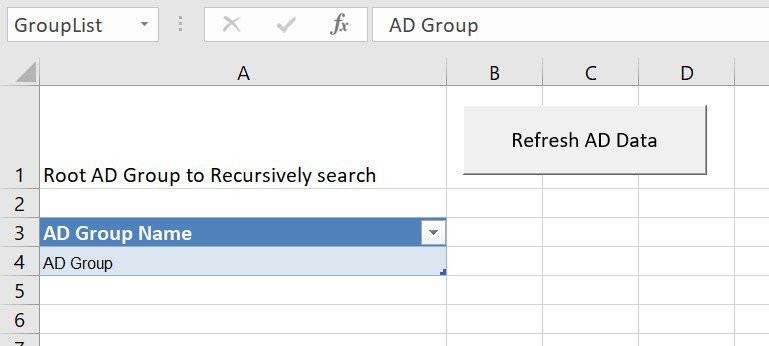Search
•MarkDataGuy

# Using PowerQuery to recursively extract data from AD

Hello,

One of the many objects that you can connect to with Power Query is Active Directory. This is very useful, especially as Active Directory is frequently the mechanism used to secure all sorts of systems, especially Analysis Services (Both Multidimensional and Tabular variations). However, what happens when you have AD groups nested within other ad groups?

In my circumstance, there is a single root AD groups called BUSINESS_ROLES, and nested within this group, there are a bunch of other AD Groups that contain users and other groups that may contain more user and more ad groups..... You can see where am I going with this! Wouldn't it be great if you could put in my root AD Group in PowerQuery and get all the users, irrespective of how deep they are nested?With Power Query, you can resolve this by writing a recursive Power Query function. In simple form, you want to pass an AD Group (or list of Groups) to a function, which iterates over that list to get each member group and get the member groups of those groups by recursively calling itself! (Yep, recursion make my head hurt)

A the end of this article, there is a link to my Github where you can download an Excel workbook that will do exactly this, however, the magic is achieved by this bit of M code.

```(ADGroup as text) as list =>
let
getad = (parent as text) as list =>
let
in
,
in
Result```

There are two functions going on here. The first is in red and repeated here;

```(ADGroup as text) as list =>
let
,
in
Result

```

This function is simple, it takes as an input, the name of an AD Group. The function returns the result of the call to the "getad" function that is declared in purple above and repeated here

```getad = (parent as text) as list =>
let
in

```

This code declares a function "getad", which takes a text as an input (the name of an ad group) and returns a list. The first part of the code

`childern = fctGetADGroupGroups(parent),`

is simple, it is a call to another PowerQuery function that returns any groups that are a member of the group name in the variable "parent". The code on how the "fctGetADGroupGroups" function works is included in my GitHub excel file.

The second bit of code is the bit that does the recursion.

`Ad = List.Combine({{parent}, List.Accumulate(childern, {}, (a , b) =>  List.Combine({a,@getad(b)}))})`

What we want to achieve is that we start with a list of a single AD group. We then get any group member of that group and add that to the list. We now have a bigger list, over which we then repeat the process, each member is checked, and any new groups are added to the list. This continues until no new members are added, and you end up with the full list of group names. This is the most complex aspect of the function, so let's break it down;

List.Combine takes a list of lists and combines them. In our case, it takes the original AD group passed in from parent and adds to that list the list returned from the List.Accumulate function.

List.Accumulate take 3 parameters. A start list, a start value, and a function to apply to each element in the start list. So the code "List.Accumulate(a, {}, (state, current) => state + current)" starts with a State of an empty set "{}". It this iterates over the set "a" via the "current" operator and applies to this iteration the result of state + current member.

So with sample code as follows;

`List.Accumulate({1, 2, 3, 4, 5}, 0, (state, current) => state + current)`

the results are;

1st Step - State = 0, current = 1, so new state = 0 + 1

2nd Step - State = 1, current = 2, so new state = 1 + 2

3rd Step - State = 3, current = 3, so new state = 3 + 3

3rd Step - State = 6, current = 4, so new state = 6 + 4

5th Step - State = 10, current = 5, so new state = 10 + 5

Result = 15 as this is the end of our list referenced in the accumulate.

So if we take our List.Accumulate code;

`Ad = List.Accumulate(childern, {}, (a , b) =>  List.Combine({a,@getad(b)}))`

We evaluate as follows;

1st Step - State {}, Current = {1st Member of AD Group List Childern}, so New State = 1st Member + Child AD Groups of 1st Member

..

1st Step of 1st Recursive Call = State {}, Current = {1st Member of 1st Member of Group} + Child AD Groups of 1st of 1st member

etc

Recursion hurts my head, but basically, you keep iterating from the original AD group until you have extracted a list of all members of each member until you hit a member that no longer has a member, and which point the recursion for that member collapses.

In my github excel book, you have the following elements in PowerQuery

ADAccountStatus - A query that retrieves from the excel workbook the descriptions of AD Account Status

Recursive AD Group Membership - The Query that returns data to the excel table

fctGetADRecursive - The recursive function that is called from "Recursive AD Group Membership" and returns all nested groups

fctGetADGroupUsers - The function that returns the user associated with the recursively generated list.

fctGetADGroupGroups - Called by the recursive function to get group member of a group

I hope you find this of help.

Mark